## RS Aggarwal Class 7 Solutions Chapter 12 Simple Interest CCE Test Paper

These Solutions are part of RS Aggarwal Solutions Class 7. Here we have given RS Aggarwal Solutions Class 7 Chapter 12 Simple Interest CCE Test Paper.

Other Exercises

Question 1.
Solution: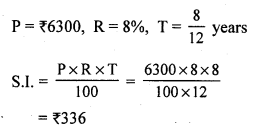Question 2.
Solution:
Let the sum be ₹ x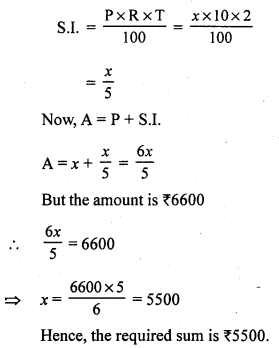Question 3.
Solution:
P = ₹ 3625, A = ₹ 4495, T = 2 years
S.I. = A – P = ₹ 4495 – ₹ 3625 = ₹ 870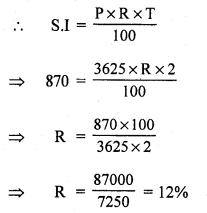Question 4.
Solution:
P = ₹ 3600, A = ₹ 4410, R = 9%
S.I. = A – P = ₹ 4410 – ₹ 3600 = ₹ 810Question 5.
Solution:
Let the sum be ₹ x
Amount = ₹ 2x
S.I. = (2x – x) = ₹ x
Time = 12 years
P = x, S.I. = x, T = 12 yearsQuestion 6.
Solution:
Let the sum be ₹ 4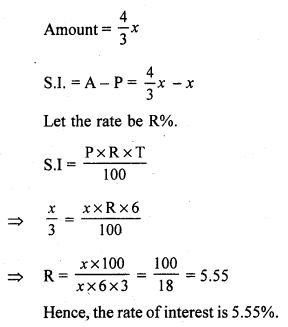Mark (✓) against the correct answer in each of the following :
Question 7.
Solution:
(c) 9%
Let the sum be ₹ x
A = $$\frac { 49x }{ 40 }$$
We know:
A = P + S.I.
S.I. = A – PQuestion 8.
Solution:
(c) ₹ 3500
A = ₹ 3626, R = 6%,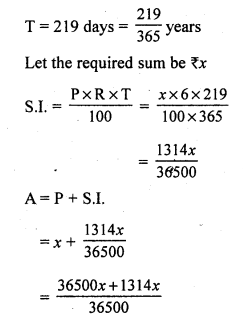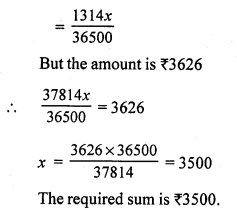Question 9.
Solution:
(a) 9 months
P = ₹ 6000, A = ₹ 6360
S.I. = A – P = 6300 – 6000 = ₹ 360Question 10.
Solution:
(c) 12%
Let the sum be ₹ x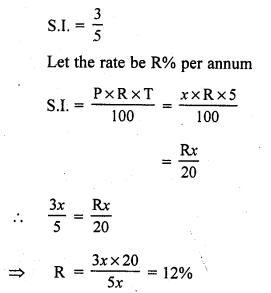Question 11.
Solution:P = ₹ $$\frac { 100 }{ x }$$

Question 12.
Solution:
(b) 10%
Let the sum be ₹ x
Amount ₹ 2x
Time =10 years
S.I. = A – P = 2x – x = ₹ x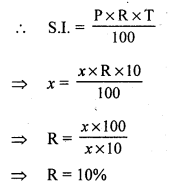Question 13.
Solution: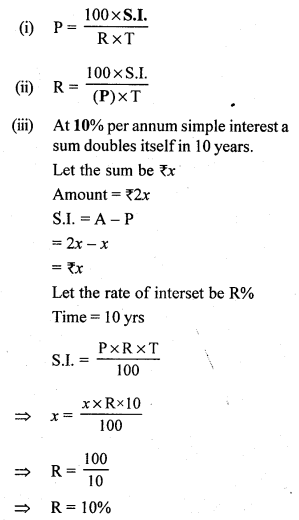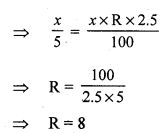Question 14.
Solution:
(i) False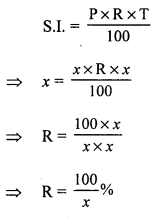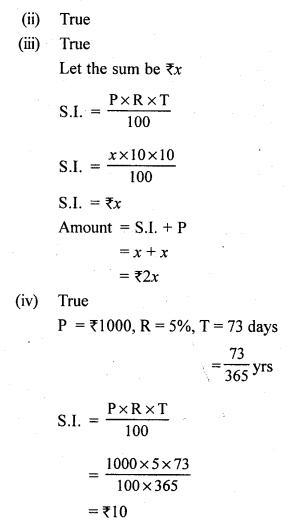Hope given RS Aggarwal Solutions Class 7 Chapter 12 Simple Interest CCE Test Paper are helpful to complete your math homework.

If you have any doubts, please comment below. Learn Insta try to provide online math tutoring for you.